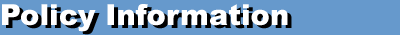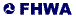An update of the manual is available! - HPMS Field Manual

 << Previous Contents Next >>

## HPMS Field Manual

### Appendix D: Sample Size Estimation Procedures

The sample size estimates for each stratum are derived from the following formula:

 n = Z2 C2 / d21 + (1/N) ((Z2 C2 / d2)- 1)
Where: n = Required sample size
Z = Value of the standard normal statistic for an alpha confidence level (two-sided):

Confidence Level Value of Z Z Squared
90 Percent 1.645 2.706
80 Percent 1.282 1.644
70 Percent 1.040 1.082
d = Desired precision rate (from Appendix C, HPMS Field Manual)
N =& Universe or population stratum size (number universe sections available for sampling in a volume group)

For example, the sample size for a stratum with a desired precision rate of "5 percent and a 90-percent confidence level, an AADT coefficient of variation of 0.40, and 300 available universe sections for sampling, is estimated by:

 n = (1.645)2 (.40)2 / (.05)21 + (1/300) ((1.645)2 (.40)2 / (.05)2) - 1) = 173.181 + (172.18/300) = 110 required samples

If the total number of sections available for sampling (N) is not known because the State groups some or all of its nonsampled universe length, an estimate of this total may be obtained by dividing the total universe volume group length by an estimate of the average section length or the average length of the existing samples in the volume group.

The critical point in this process is the value designation of C, the AADT coefficient of variation. The original HPMS design was based on empirical estimates using data from a small number of States. The procedures presented here require the estimation of AADT coefficients of variation based on the latest State data. The results are then always up to date, based on the latest information, and are tailored to the specific State.

Estimates of the AADT coefficients of variation for a particular State can be derived from its existing HPMS data using standard statistical computer packages. The HPMS software generates the AADT coefficients of variation from a State's universe and sample data using standard statistical procedures, and produces reports that enable the State to analyze and review either the HPMS standard sample panel or the nonattainment or maintenance area supplementary (donut) sample panel.

 << Previous Contents Next >>

Updated: 06/30/2017United States Department of Transportation - Federal Highway Administration